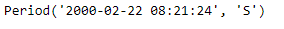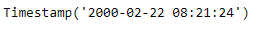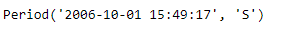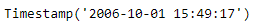# Python | Pandas Period.start_time

• Last Updated : 06 Jan, 2019

Python is a great language for doing data analysis, primarily because of the fantastic ecosystem of data-centric python packages. Pandas is one of those packages and makes importing and analyzing data much easier.

Pandas` Period.start_time` attribute returns a Timestamp object containing the start time of the given period object.

Attention geek! Strengthen your foundations with the Python Programming Foundation Course and learn the basics.

To begin with, your interview preparations Enhance your Data Structures concepts with the Python DS Course. And to begin with your Machine Learning Journey, join the Machine Learning - Basic Level Course

Syntax : Period.start_time

Parameters : None

Return : Timestamp object

Example #1: Use `Period.start_time` attribute to find the start time for the given Period object.

 `# importing pandas as pd``import` `pandas as pd`` ` `# Create the Period object``prd ``=` `pd.Period(freq ``=``'S'``, year ``=` `2000``, month ``=` `2``, day ``=` `22``,``                         ``hour ``=` `8``, minute ``=` `21``, second ``=` `24``)`` ` `# Print the Period object``print``(prd)`

Output :Now we will use the `Period.start_time` attribute to find the start time for prd object.

 `# return the start time``prd.start_time`

Output :As we can see in the output, the `Period.start_time `attribute has returned a Timestamp object which contains the date value as well as the start time of the given period object.

Example #2: Use `Period.start_time` attribute to find the start time for the given Period object.

 `# importing pandas as pd``import` `pandas as pd`` ` `# Create the Period object``prd ``=` `pd.Period(freq ``=``'S'``, year ``=` `2006``, month ``=` `10``, ``               ``hour ``=` `15``, minute ``=` `49``, second ``=` `17``)`` ` `# Print the object``print``(prd)`

Output :Now we will use the `Period.start_time` attribute to find the start time for prd object.

 `# return the start time``prd.start_time`

Output :As we can see in the output, the `Period.start_time `attribute has returned a Timestamp object which contains the date value as well as the start time of the given period object.

My Personal Notes arrow_drop_up